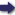(London :  Kegan Paul, Trench, Trübner & Co.,  1910.)

 Tools

## Search this bookPrev Page 314 Next``` 314 ALBERUNPS INDIA. these day-minutes by the circumference of the earth, and divides the product by 6o, viz. the minutes (or 6oth parts) of the daily revolution. The quotient, then, is the number of the yojanas of the distance between the two places. This calculation is correct. The result refers to the great circle on which Lahka lies. Brahmagupta calculates in the same manner, save that he multiplies by 4800. The other details have already been mentioned. Calculation As far as this, one clearly recognises what the Hindu deidn^tara astrouomers aim at, be their method correct or faulty, Aifazart^ ° Howcvcr, WO caunot say the same of their calculation of the desdntara from the latitudes of two different places, which is reported by Alfazari in his canon in the fol¬ lowing manner :— " Add together the squares of the sines of the lati¬ tudes of the two places, and take the root of the sum. This root is the portio. "Further, square the difference of these two sines and add it to the portio. Multiply the sum by 8 and divide the product by 377, The quotient, then, is the distance between the two places, that is to say, according to a rough calculation. " Further, multiply the difference between the two latitudes by the yojctnas of the circumference of the earth and divide the product by 360." Evidently this latter calculation is nothing but the transferring of the difference between the two latitudes from the measure of degrees and minutes to the mea¬ sure of yojanas. Then he proceeds :— " Now the square of the quotient is subtracted from the square of the roughly calculated distance, and of the remainder you take the root, which represents the straight yojanas.'" Page 162, Evidently the latter number represents the distance between the spheres of the meridians of the two places ```Prev Page 314 Next